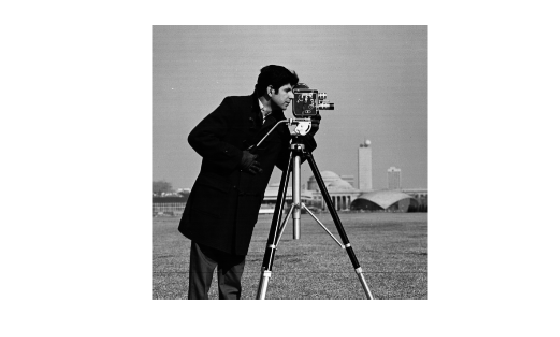edgetaper

Taper discontinuities along image edges

Description

example

J = edgetaper(I,PSF) blurs the edges of the input image I using the point spread function PSF.

The output image J is the weighted sum of the original image I and its blurred version. The weighting array, determined by the autocorrelation function of PSF, makes J equal to I in its central region, and equal to the blurred version of I near the edges.

The edgetaper function reduces the ringing effect in image deblurring methods that use the discrete Fourier transform, such as deconvwnr, deconvreg, and deconvlucy.

Examples

collapse all

PSF = fspecial('gaussian',60,10);
edgesTapered = edgetaper(original,PSF);
figure, imshow(original,[]);figure, imshow(edgesTapered,[]);Input Arguments

collapse all

Input image, specified as a numeric array.

Data Types: single | double | int16 | uint8 | uint16

Point spread function, specified as a numeric array. The size of the PSF cannot exceed half of the image size in any dimension.

Data Types: single | double | int16 | uint8 | uint16

Output Arguments

collapse all

Weighted sum of original image and its blurred version, returned as a numeric array the same size and class as I. The weighting array, determined by the autocorrelation function of PSF, makes J equal to I in its central region, and equal to the blurred version of I near the edges.